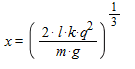# Seperation between two charged ball

## Homework Statement

Two identical insulating balls of mass m hang from massless strings of length l and carry identical electric charges, q. you may assume that the angle of separation θ is so small that tanθ ≅sinθ≅θ.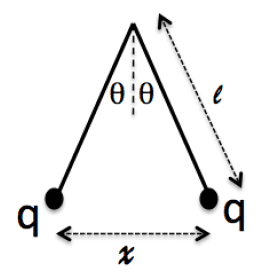What is the separation distance between the two masses x?

## The Attempt at a Solution

I made two attempts at a solution, though it has been so long since I worked a problem like this that I don't know if either attempt is correct (I do know that both methods give different values for x, so at least one is incorrect).

1st using energy conservation: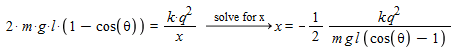I believe this method gives the incorrect answer as there is some initial energy between the two particles I have not accounted for, when the potential energy is zero but the energy from the E-field is at its greatest. Because I do not know the diameter of the balls, or their starting position, I assume this method is a bad one?

2nd method using statics: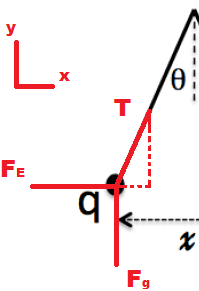Based off the free body diagram...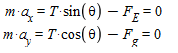thus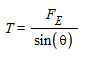so then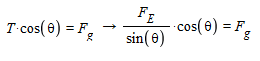where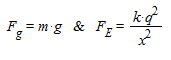as such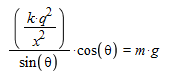and finally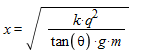Is this correct? Can someone please point me in the right direction?

#### Attachments

Last edited:

mfb
Mentor
I believe this method gives the incorrect answer as there is some initial energy between the two particles I have not accounted for, when the potential energy is zero but the energy from the E-field is at its greatest.
Right. You don't know the total energy in the system, and the particles being at the same place is an unphysical situation.
2nd method using statics:
Shouldn't there be some equations in this part?

Right. You don't know the total energy in the system, and the particles being at the same place is an unphysical situation.Shouldn't there be some equations in this part?
My images did not upload. Give me a second... Sorry.

Edit: I've fixed it now. Not sure why it didn't work the first time...

Chandra Prayaga
Was there any reson for you to doubt your second method?

Was there any reson for you to doubt your second method?

The problem mentioned that I should be aware of the small angle approximation, which I did not use. Also, I was uncertain if I would have to account for the second ball in this problem. I feel like I had already done so, but I wanted to be certain of that.

mfb
Mentor
You expressed x as function of the angle (and other constants). The answer should not depend on the unknown angle. If you could use the angle in the answer, simple geometry would be much faster.

You expressed x as function of the angle (and other constants). The answer should not depend on the unknown angle. If you could use the angle in the answer, simple geometry would be much faster.

Yes, you could just take the sin of that angle, and multiply it by twice the length of the string.

So is there a better way to do this? Is there a way to do this without knowing the final angle of separation or the distances between the particles?

Chandra Prayaga
Notice that the final result you got contains both x and θ. You can eliminate one of them simply in the small angle approximation. You can use:

tanθ≈sinθ=x/l

and then solve for x in terms of just the mass and charge of each ball.

Notice that the final result you got contains both x and θ. You can eliminate one of them simply in the small angle approximation. You can use:

tanθ≈sinθ=x/l

and then solve for x in terms of just the mass and charge of each ball.
Ah, so then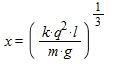would be the final answer in terms of everything we "know"?

#### Attachments

ehild
Homework Helper
Notice that the final result you got contains both x and θ. You can eliminate one of them simply in the small angle approximation. You can use:

tanθ≈sinθ=x/l
No, sinθ=x/(2l)

haruspex
Homework Helper
Gold Member
Ah, so then View attachment 229589 would be the final answer in terms of everything we "know"?
Not quite, because
sinθ=x/l
Should be sinθ=x/(2l)

... beaten to it by ehild.

Chandra Prayaga